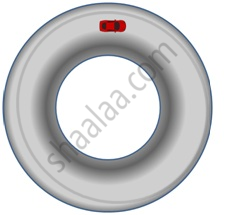Share

# Circular Motion

Course

## Circular motion

• Motion in a circular path

• Centripetal force plays a crucial role in circular motion.

• It is the Force that makes a body follow a curved path. It acts towards the centre.Generally denoted by Fc

• Fc= (mv^2)/r

Since we know that F = ma; therefore (v^2) /r is termed as Centripetal acceleration

S